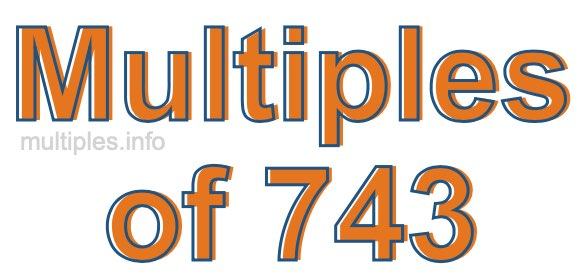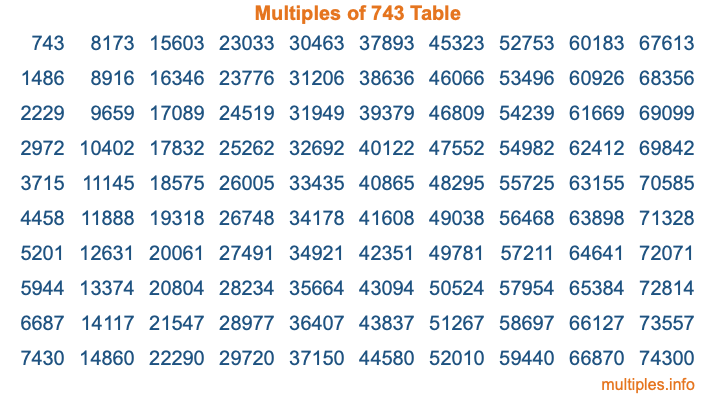Multiples of 743Welcome to the Multiples of 743 page. Here we will first teach you everything you will ever need to know about the multiples of 743, and then give you a study guide summary of everything we taught you to make sure you remember it all. Use this page to look up facts and learn information about the multiples of 743. This page will make you a multiples of seven hundred forty-three expert!

Definition of Multiples of 743
Multiples of 743 are all the numbers that when divided by 743 equal an integer. Each of the multiples of 743 are called a multiple. A multiple of 743 is created by multiplying 743 by an integer.

Therefore, to create a list of multiples of 743, you start with 1 multiplied by 743, then 2 multiplied by 743, then 3 multiplied by 743, and so on for as long as you want. Thus, the list of the first five multiples of 743 is 743, 1486, 2229, 2972, and 3715. To see a larger list of multiples of 743, see the printable image of Multiples of 743 further down on this page. We also have a category where you can choose any nth multiple of 743.

Multiples of 743 Checker
The Multiples of 743 Checker below checks to see if any number of your choice is a multiple of 743. In other words, it checks to see if there is any number (integer) that when multiplied by 743 will equal your number. To do that, we divide your number by 743. If the the quotient is an integer, then your number is a multiple of 743.

Is  a multiple of 743?

Least Common Multiple of 743 and ...
A Least Common Multiple (LCM) is the lowest multiple that two or more numbers have in common. This is also called the smallest common multiple or lowest common multiple and is useful to know when you are adding our subtracting fractions. Enter one or more numbers below (743 is already entered) to find the LCM.

Check out our LCM Calculator if you need more details about the Least Common Multiple or if you need the LCM for different numbers for adding and subtraction fractions.

nth Multiple of 743
As we stated above, 743 is the first multiple of 743, 1486 is the second multiple of 743, 2229 is the third multiple of 743, and so on. Enter a number below to find the nth multiple of 743.

th multiple of 743

Multiples of 743 vs Factors of 743
743 is a multiple of 743 and a factor of 743, but that is where the similarities end. All postive multiples of 743 are 743 or greater than 743. All positive factors of 743 are 743 or less than 743.

Below is the beginning list of multiples of 743 and the factors of 743 so you can compare:

Multiples of 743: 743, 1486, 2229, 2972, 3715, etc.

Factors of 743: 1, 743

As you can see, the multiples of 743 are all the numbers that you can divide by 743 to get a whole number. The factors of 743, on the other hand, are all the whole numbers that you can multiply by another whole number to get 743.

It's also interesting to note that if a number (x) is a factor of 743, then 743 will also be a multiple of that number (x).

Multiples of 743 vs Divisors of 743
The divisors of 743 are all the integers that 743 can be divided by evenly. Below is a list of the divisors of 743.

Divisors of 743: 1, 743

The interesting thing to note here is that if you take any multiple of 743 and divide it by a divisor of 743, you will see that the quotient is an integer.

Multiples of 743 Table
Below is an image of the first 100 multiples of 743 in a table. The table is in chronological order, column by column. The first column has the first ten multiples of 743, the second column has the next ten multiples of 743, and so on.The Multiples of 743 Table is also referred to as the 743 Times Table or Times Table of 743. You are welcome to print out our table for your studies.

Negative Multiples of 743
Although not often discussed or needed in math, it is worth mentioning that you can make a list of negative multiples of 743 by multiplying 743 by -1, then by -2, then by -3, and so on, to get the following list of negative multiples of 743:

-743, -1486, -2229, -2972, -3715, etc.

Multiples of 743 Summary
Below is a summary of important Multiples of 743 facts that we have discussed on this page. To retain the knowledge on this page, we recommend that you read through the summary and explain to yourself or a study partner why they hold true.

There are an infinite number of multiples of 743.

A multiple of 743 divided by 743 will equal a whole number.

743 divided by a factor of 743 equals a divisor of 743.

The nth multiple of 743 is n times 743.

The largest factor of 743 is equal to the first positive multiple of 743.

743 is a multiple of every factor of 743.

743 is a multiple of 743.

A multiple of 743 divided by a divisor of 743 equals an integer.

743 divided by a divisor of 743 equals a factor of 743.

Any integer times 743 will equal a multiple of 743.

Multiples of a Number
Here you can get the multiples of another number, all with the same attention to detail as we did for multiples of 743 on this page.

Multiples of
Multiples of 744
Did you find our page about multiples of seven hundred forty-three educational? Do you want more knowledge? Check out the multiples of the next number on our list!